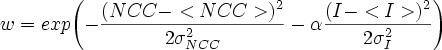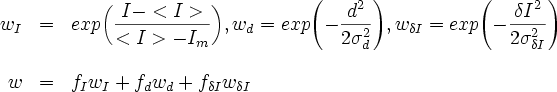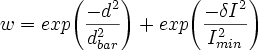## Models and Parameters:

Brief description of the models and adjustable parameters.

#### Diffusing NCC:

This model is an adaptive model that builds a template and uses a Normalized Cross Correlation filter to determine the position of the next speckle mark. The frame after the last frame of the speckle track, being tracked, is filtered with an NCC filter. Then a Square Search region is searched for the highest NCC value. That point is then checked to see if it is acceptable based on the following weight:
######.
The point is added to the track if w>wmin. The intensity is the average intensity within a circle with a radius "Inner Radius," centered at candidate position. This is how the intensity is calculated in other models.
##### Parameters

alpha: adjusts the dependence on the intensity as compared to the correlation value (see equation above).

Search Size (px): the size of one side of the box the model searches for the next speckle.

wmin: Threshold value.

#### Constant Velocity NCC:

This model uses the same criteria as the Diffusing NCC model except it assumes the particles move with a constant velocity. The expected velocity is calculated by fitting each existing speckle track to a line. The slope of the line is the velocity.

See above.

#### Diffusing Spots:

This is similar to the Diffusing NCC model except that it does not use correlations. It relies on intensity, changes in intensity and local maxima. The weight function is as follows.
######δI is the change in intensity from the previous speckle mark to the candidate speckle mark.
##### Parameters

fI: determines the contribution of the Intensity to the total weight.

fd: determines the contribution of the displacement to the total weight.

fδI: determines the contribution of the change in intensity to the total weight.

Search Size (px): the size of one side of the box the model searches for the next speckle.

wmin: minimum weight value that for an acceptable mark.

#### Diffusing Spots Backward:

The Diffusing Spots model, but it moves through frames backwards in time. Both models share the same parameters.

See above.

#### Fixed Parameters Diffusing Spots:

Searches for candidate speckle marks the same as the Diffusing Spots model. The displacement and the relative change in intensity are used to sort the cadidate positions using the following function:
######The best point is accepted if I>Imin. The intensity, I, is the average intensity over a circle with the global parameter "inner radius."
##### Parameters

Imin: minimum average intensity that determines if a location is acceptable for a speckle mark.

dbar: average distance that the particle is expected to move.

Search Size (px): the size of one side of the box the model searches for the next speckle.

#### Static:

This model creates a speckle mark in every frame. The position of the new marks depend on the position of the existing speckle marks. For frames before the first existing mark, the new speckle marks are placed at the position of the first speckle mark. For frames after the last speckle mark, the new marks are placed at the position of the last speckle mark.

None.

#### Extend Linear Refine:

Similar to the static model, this model will create a speckle mark in every frame. This model applies the center of intensity algorithm starting at the location of the previous speckle mark for five iterations or until the change is smaller than 0.01 px. After all marks have been placed a least squares fit is used to refine the track as in the Linear Refine model (separately for each track). Thus it produces a straight track from the beginning of the movie until the end that moves with a small velocity.
##### Parameters

None.

This model applies the center of intensity algorithm to each speckle mark. The position is updated by finding the center of intensity over a 5x5 square centered at the speckle mark. The algorithm is applied five times or until the displacement is less than 0.01 px.

None.

#### Linear Refine:

This model applies the center of intensity algorithm to each speckle mark, same as in the Adjustment Model above. Then this model performs a weighted least squares fit to the positions of the speckle track to obtain a velocity. Each point is weighted proportionally to the local intensity. All marks are updated to lie on a straight line trajectory determined by this velocity. Each track has its own velocity. The model also fills missing marks: for example if a speckle track had a mark in frame 3 and a mark in frame 5 then after applying the Linear Refine model there would be a mark in frame 4.

#### Gaussian Fit:

This model fits a 2d Gaussian to the intensity of a square ( default value: 11x11 px2 ) near a speckle mark by finding the best least squares fit. The fit is started with a user-defined standard deviation of the Gaussian, sigma (1 px is the default value). The position of the center of the Gaussian is varied using a variant of the simplex method as follows. Four points are picked along the x and y axes with a specified space step (default value: 0.75 px) from the originally-estimated position. The best fit amplitude and background of a 2D Gaussian centered at each point is found using a linear least squares fit, and the error is calculated. The results are sorted according to the error, which is the sum of the squares of residuals. The point with the highest error is moved toward the point with the lowest error, at the midpoint position. The process of moving the point with the highest error is iterated and continues until the largest difference in error among the points is below a threshold value (10 -6). This results in an estimate of the best position. The whole process is repeated for different sigma, using a 1D golden section algorithm with initial step dsigma = 0.1 px to select for the sigma that minimizes the error. The fit is finished when the change disgma is sufficiently small (less than 0.02 px).
##### Parameters

Initial Sigma (px): Initial guess for a sigma value.

Maximum Sigma (px): If sigma is larger than this value the scan is stopped.

Minimum Sigma (px): If sigma goes lower than this the scan is stopped.

Initial Space Step (px): Determines the size of the space explored for the center of the Gaussian.

Fit Region Size (px): Half width of the size of square used for fitting the Gaussian.

#### Filler:

This model is for filling in discontinuous tracks, without modifying the existing marks. The new marks connect the old marks along a straight line.

### 2) Global Parameters

If the parameter name has "Batch Locate" then it only affects the batch locate routine.

Inner Radius The red line on the profiler is average intensity for a circle of this radius. This radius is also used by models to calculate the intensity.

Outer Radius The blue line on the profiler is the average intensity of a ring whose outer radius is determined by this parameter.

NCC Template Size The size of a square region used to create a template for Normalized Cross Correlation analysis. This value needs to be an odd number. The NCC models and Template Locate use this value.

Minimum Track Separation When detecting candidates this is the minimum distance to the nearest neighboring candidate. If a candidate is closer than this distance the smaller candidate is removed. When tracking multiple speckles this value is the minimum distance new speckle marks can be placed. If paths cross, then the track with the lower 'weight' mark, as determined by the model, is stopped from continuing.

Batch Locate Start Frame The first frame that the batch locate routine starts from.

Batch Locate Ending Frame Last frame to try and detect speckles. Speckle tracks can extend beyond this frame.

Batch Locate Threshold Value The intensity threshold value used for detecting speckles. This value can be set by clicking "learn" or the "acquire" button from the Batch Locate dialog.

Batch Locate Minimum Detection Size Minimum number of pixels of a connected component that is accepted after the connected component algorithm has identified speckle candidates.

Batch Locate Minimum Duration The minimum number of frames that a speckle track needs to exist to be accepted during batch locate. After a speckle has been detected and tracked, the duration of the speckle track is checked and the track is removed if it is too short.

Batch Locate Fit Tracks to Line After a speckle has been tracked, during batch locate, it is refined. This parameter determines which model is used to refine the speckle track. If the value is one then the speckle track will be refined with the "Linear Refine" model. If this parameter is zero, then the speckle track will be refined with the "Adjustment" model.

Batch Locate Maximum Mean Displacement After a speckle track has been refined, if the average displacement per frame (distance between the first frame and the last frame divided by the track length) is too large, the speckle track is removed. This value is px per frame.

Batch Locate Only Fusion If this is greater than zero, then speckles tracks are removed if they are determined not to be a vesicle fusion event.

Batch Locate Link Frames After a speckle track has been accepted, the program checks for speckle tracks that ended nearby, within the "Minimum Track Separation," in a frame previous to the start of the current speckle track. If a speckle track meets this requirement then the two tracks are merged. This parameter is the number of frames, prior to the start of the current speckle track, that are checked. If multiple tracks meet this criteria, then the one that ends in the frame closest to the starting frame of the current speckle track is accepted.